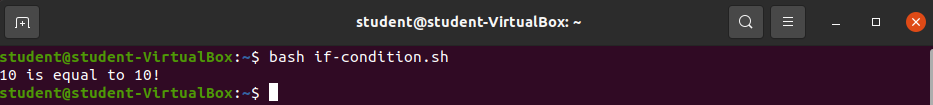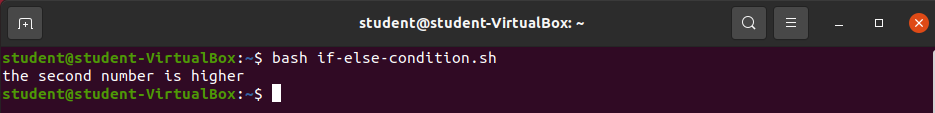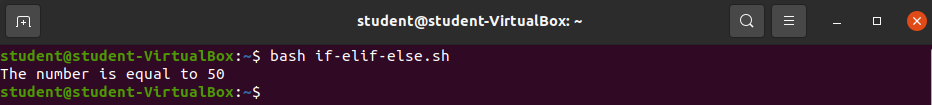# Conditionals

## Conditional Statements in Bash

Similar to many programming languages `bash` allows you to write conditional statements to control the flow of a script:

``````if [[ condition ]]
then
clause statement
fi
``````
``````if condition:
clause statement
``````
``````if (condition) {
clause statement
}
``````
``````if (condition) {
clause statement
}
``````

`Bash` requires a closing “`fi`” for `if` statements.

## Operators

Bash has built in binary operators for the following:

• equal to: `-eq`
• not equal to: `-ne`
• less than: `-lt`
• less than or equal to: `le`
• greater than: `-gt`
• greater than or equal to: `-ge`

Some of the above operators will be used in the following walkthroughs for the `Bash: Scripting` chapter.

## Examples

### if Statement

Create a new file called `if-condition.sh`.

``````#!/bin/bash

number1=10

if [[ \$number1 -eq 10 ]]
then
echo "\$number1 is equal to 10!"
fi
``````

Save the above code to the `if-condition.sh` file and exit the editor.

Run the command `bash if-condition.sh`.### if else statement

Create a new file called `if-else-condition.sh`.

``````#!/bin/bash

number1=1
number2=2

if [[ \$number1 -gt \$number2 ]]
then
echo "The first number is higher"
else
echo "The second number is higher"
fi
``````

Save the above code to the `if-else-condition.sh` file and exit the editor.

Run the command `bash if-else-condition.sh`.### if elif else statement

Create a new file called `if-elif-else.sh`.

``````#!/bin/bash

new_number=50

if [[ \$new_number -lt 50 ]]
then
echo "The number is less than 50"
elif [[ \$new_number -eq 50 ]]
then
echo "The number is equal to 50"
else
echo "The number is greater than 50"
fi
``````

Save the above code to the `if-elif-else.sh` file and exit the editor.Run the command `bash if-elif-else.sh`.

Try changing the value of `new_number`!

## Recap

• `if` statement syntax
• `if` statement example
• `if else` example
• `if elif else` example
• `fi`: closing if statement